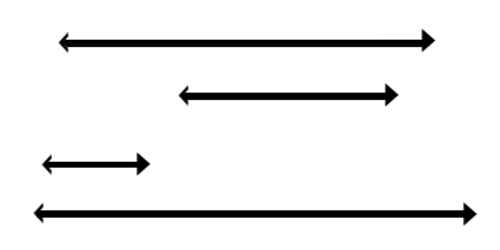Mathematic

# Co-Ordinate Straight Line GeometryCo-Ordinate Straight Line Geometry

A line is simply an object in geometry that is characterized as a straight, thin, one-dimensional, zero width object that extends on both sides to infinity. A straight line is essentially just a line with no curves. Most of the time, when we speak about lines, we are talking about straight lines! Here are some examples of straight lines

The intercepts of a line are the points where the line intercepts, or crosses, the horizontal and vertical axes. A straight line moves so that the sum of the reciprocals of its intercepts made on axes is constant.

(i) The slope or gradient of a straight line is the trigonometric tangent of the angle θ which the line makes with the positive directive of x-axis.

(ii) The slope of x-axis or of a line parallel to x-axis is zero.

(iii) The slope of y-axis or of a line parallel to y-axis is undefined.

(iv) The slope of the line joining the points (x1, y1) and (x2, y2) is

m = (y2 – y1)/(x2 – x1).

(v) The equation of x-axis is y = 0 and the equation of a line parallel to x-axis is y = b.

(vi) The equation of y-axis is x = 0 and the equation of a line parallel to y-axis is x = a.

(vii) The equation of a straight line in

• slope-intercept form: y = mx + c where m is the slope of the line and c is its y-intercept;
• point-slope form: y – y1 = m (x – x1) where m is the slope of the line and (x1 , y1) is a given point on the line;
• symmetrical form: (x – x1)/cos θ = (y – y1)/sin θ = r, where θ is the inclination of the line, (x1, y) is a given point on the line and r is the distance between the points (x, y) and (x1, y1);
• two-point form: (x – x1)/(x2 – x1) = (y – y1)/(y2 – y1) where (x1, y1) and (x2, y2) are two given points on the line;
• intercept form: x/a + y/b = 1 where a = x-intercept and b = y-intercept of the line;
• normal form: x cos α + y sin α = p where p is the perpendicular distance of the line from the origin and α is the angle which the perpendicular line makes with the positive direction of the x-axis.
• general form: ax + by + c = 0 where a, b, c are constants and a, b are not both zero.

(viii) The equation of any straight line through the intersection of the lines a1x + b1y + c1 = 0 and a2x + b2y + c2 = 0 is a1x + b1y + c + k(a2x + b2y + c2) = 0 (k ≠ 0).

(ix) If p ≠ 0, q ≠ 0, r ≠ 0 are constants then the lines a1x + b1y + c1 = 0, a2x + b2y + c2 = 0 and a3x + b3y + c3 = 0 are concurrent if P (a1x + b1y + c1) + q (a2x + b2y + c2) + r (a3x + b3y + c3) = 0.

(x) If θ be the angle between the lines y= m1x + c and y = m2x + c2 then tan θ = ± (m1 – m2)/(1 + m1 m2);

(xi) The lines y= m1x + c1 and y = m2x + c2 are

(a) parallel to each other when m1 = m2;

(b) perpendicular to one another when m1 ∙ m2 = – 1.

(xii) The equation of any straight line which is

(a) parallel to the line ax + by + c = 0 is ax + by = k where k is an arbitrary constant;

(b) perpendicular to the line ax + by + c = 0 is bx – ay = k1 where k1 is an arbitrary constant.

(xiii) The straight lines a1x + b1y + c1 = 0 and a2x + b2y + c2 = 0 are identical if a1/a2 = b1/b2 = c1/c2.

(xiv) The points (x1, y1) and (x2, y2) lie on the same or opposite sides of the line ax + by + c = 0 according as (ax1 + by + c) and (ax2 + by2 + c) are of same sign or opposite signs.

(xv) Length of the perpendicular from the point (x1, y1) upon the line ax + by + c = 0 is|(ax1 + by1 + c)|/√(a2 + b2).

Information Source: